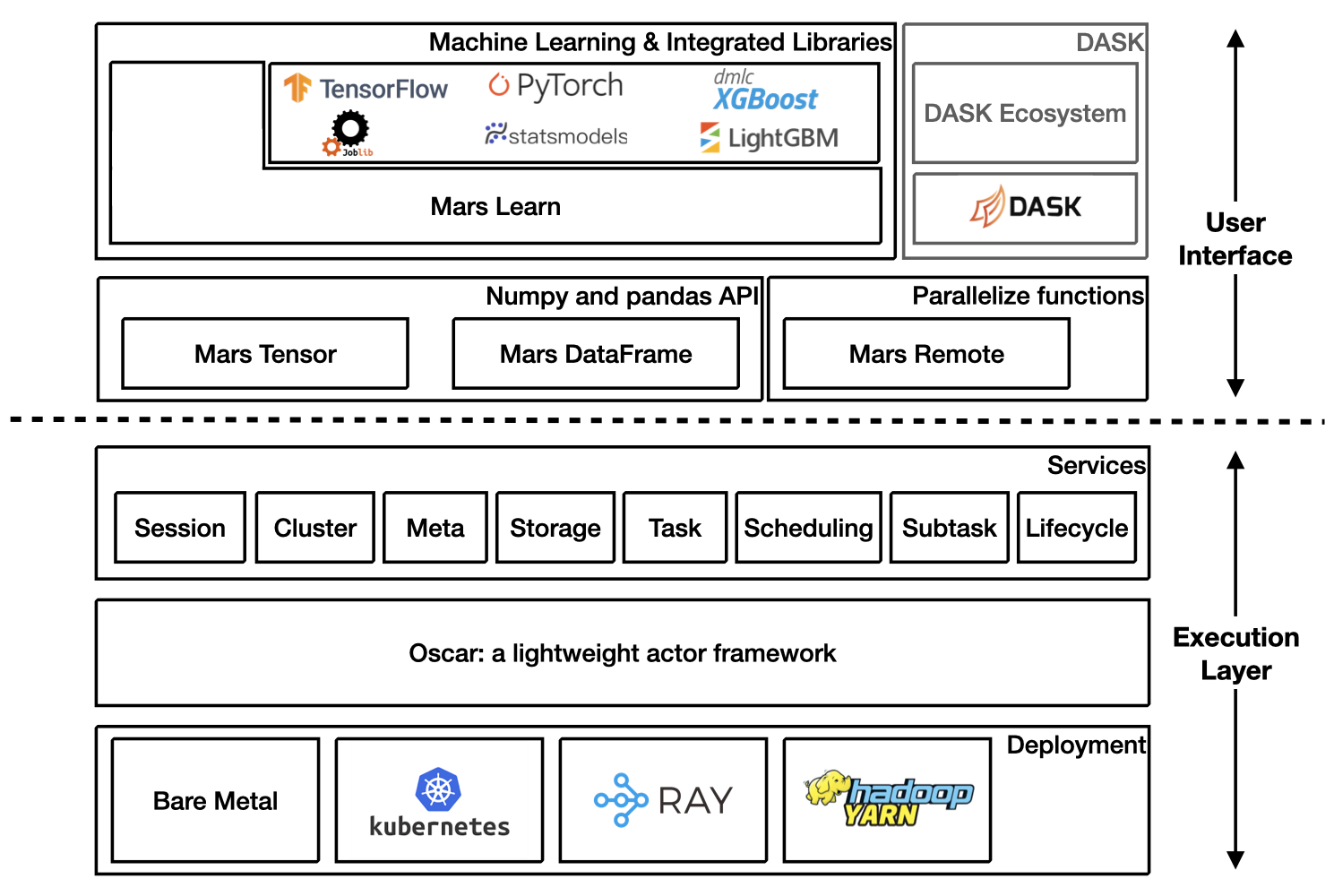# Mars Documentation#

Mars is a tensor-based unified framework for large-scale data computation which scales numpy, pandas, scikit-learn and many other libraries.

## Architecture Overview### Getting Started#

Starting a new runtime locally via:

```>>> import mars
>>> mars.new_session()
```

Or connecting to a Mars cluster which is already initialized.

```>>> import mars
>>> mars.new_session('http://<web_ip>:<ui_port>')
```

## Mars tensor#

documentation

Mars tensor provides a familiar interface like Numpy.

 Numpy Mars tensor ```import numpy as np N = 200_000_000 a = np.random.uniform(-1, 1, size=(N, 2)) print((np.linalg.norm(a, axis=1) < 1) .sum() * 4 / N) ``` ```import mars.tensor as mt N = 200_000_000 a = mt.random.uniform(-1, 1, size=(N, 2)) print(((mt.linalg.norm(a, axis=1) < 1) .sum() * 4 / N).execute()) ``` ```3.14174502 CPU times: user 11.6 s, sys: 8.22 s, total: 19.9 s Wall time: 22.5 s ``` ```3.14161908 CPU times: user 966 ms, sys: 544 ms, total: 1.51 s Wall time: 3.77 s ```

Mars can leverage multiple cores, even on a laptop, and could be even faster for a distributed setting.

## Mars dataframe#

documentation

Mars DataFrame provides a familiar interface like pandas.

 Pandas Mars DataFrame ```import numpy as np import pandas as pd df = pd.DataFrame( np.random.rand(100000000, 4), columns=list('abcd')) print(df.sum()) ``` ```import mars.tensor as mt import mars.dataframe as md df = md.DataFrame( mt.random.rand(100000000, 4), columns=list('abcd')) print(df.sum().execute()) ``` ```CPU times: user 10.9 s, sys: 2.69 s, total: 13.6 s Wall time: 11 s ``` ```CPU times: user 1.21 s, sys: 212 ms, total: 1.42 s Wall time: 2.75 s ```

## Mars learn#

documentation

Mars learn provides a familiar interface like scikit-learn.

 Scikit-learn Mars learn ```from sklearn.datasets import make_blobs from sklearn.decomposition import PCA X, y = make_blobs( n_samples=100000000, n_features=3, centers=[[3, 3, 3], [0, 0, 0], [1, 1, 1], [2, 2, 2]], cluster_std=[0.2, 0.1, 0.2, 0.2], random_state=9) pca = PCA(n_components=3) pca.fit(X) print(pca.explained_variance_ratio_) print(pca.explained_variance_) ``` ```from mars.learn.datasets import make_blobs from mars.learn.decomposition import PCA X, y = make_blobs( n_samples=100000000, n_features=3, centers=[[3, 3, 3], [0, 0, 0], [1, 1, 1], [2, 2, 2]], cluster_std=[0.2, 0.1, 0.2, 0.2], random_state=9) pca = PCA(n_components=3) pca.fit(X) print(pca.explained_variance_ratio_) print(pca.explained_variance_) ```

Mars learn also integrates with many libraries:

## Mars remote#

documentation

Mars remote allows users to execute functions in parallel.

 ```import numpy as np def calc_chunk(n, i): rs = np.random.RandomState(i) a = rs.uniform(-1, 1, size=(n, 2)) d = np.linalg.norm(a, axis=1) return (d < 1).sum() def calc_pi(fs, N): return sum(fs) * 4 / N N = 200_000_000 n = 10_000_000 fs = [calc_chunk(n, i) for i in range(N // n)] pi = calc_pi(fs, N) print(pi) ``` ```import numpy as np import mars.remote as mr def calc_chunk(n, i): rs = np.random.RandomState(i) a = rs.uniform(-1, 1, size=(n, 2)) d = np.linalg.norm(a, axis=1) return (d < 1).sum() def calc_pi(fs, N): return sum(fs) * 4 / N N = 200_000_000 n = 10_000_000 fs = [mr.spawn(calc_chunk, args=(n, i)) for i in range(N // n)] pi = mr.spawn(calc_pi, args=(fs, N)) print(pi.execute().fetch()) ``` ```3.1416312 CPU times: user 32.2 s, sys: 4.86 s, total: 37.1 s Wall time: 12.4 s ``` ```3.1416312 CPU times: user 616 ms, sys: 307 ms, total: 923 ms Wall time: 3.99 s ```